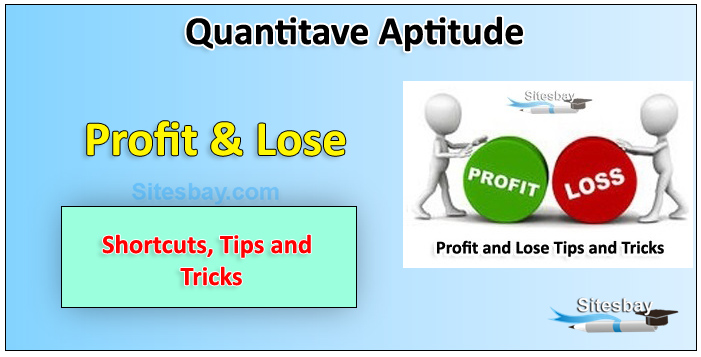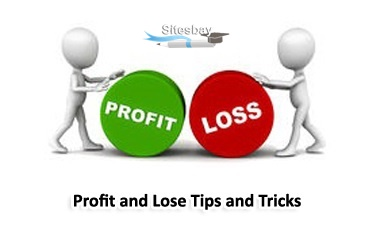# Profit and Lose Problems

## Profit and Lose Tips and Tricks

If the selling price of a product is more than the cost price, there will be profit in the deal. If the selling price of a product is less than the cost price, the seller will incur a lossCost Price: The price, at which an article is purchased, is called its cost price, abbreviated as C.P.

Selling Price: The price, at which an article is sold, is called its selling prices, abbreviated as S.P.

Profit or Gain: If S.P. is greater than C.P., the seller is said to have a profit or gain.

Loss: If S.P. is less than C.P., the seller is said to have incurred a loss.### Important Profit and Lose Formula

• Gain = (S.P.) - (C.P.)
• Loss = (C.P.) - (S.P.)
• Loss or gain is always reckoned on C.P.
• Gain Percentage: (Gain %)= Gain x 100/C.P
• Loss Percentage: (Loss %) = Loss x 100/C.P
• If an article is sold at a gain of say 35%, then S.P. = 135% of C.P.
• If an article is sold at a loss of say, 35% then S.P. = 65% of C.P.
• When a person sells two similar items, one at a gain of say x% and the other at a loss of say x%. then the seller always incurs a loss given by:
Loss% = (x/10)2
• If a seller sells his goods at cost price but uses false weights, then
Gain% = [Error/(True value - Error) * 100]%
• S.P = (100+ Gain %) /100 * (C.P)
• S.P = (100-Loss %) / 100 * (C.P)
• C.P = 100 / (100 + Gain %)* (S.P)
• C.P = 100 / (100-Loss %)* (S.P)

Rule: If a grocer sells its goods at cost price, but uses a false weigt instead of true weight. Then Gain percentage will be

## Formula

% Gain = (True Weight - False Weight) / False Weight X 100

Rule: If a shopkeeper sells his goods at x % loss on cost price but uses y gm instead of z gm, then his % profit is

## Formula

% profit is : [100+X]* Z/Y - 100

Rule: If a shopkeeper sells his goods at x % gain on cost price but uses y gm instead of z gm, then his % lose is

## Formula

% lose is : [100-X]* Z/Y - 100

Rule: If a businessman marks his goods at x % above his cost prie and allowed purchasers a discount of y % for cash, then % proft or % loss according to + ve or - ve sign is will be

## Formula

[X-Y-XY/100]%

### Profit and Lose Tricks

• Profit = (S.P.) > (C.P.)
• Loss = (S.P.) < (C.P.)
• If profit earned by selling an article is 25 %, then S.P. = 125% of C.P
• If an article is sold at a loss of 30 %, then S.P. = 70 % of C.P.
• C.P. of X = S.P. of Y